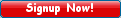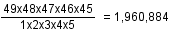How to calculate the odds of winning the jackpo Everyone wants to know their chances of winning a big prize. Odds Calculator makes it easy. Here's how it works: The odds of winning the jackpot of a lotto game are determined by the game's possible number combinations. Consider a 6/49 lotto game. There are six numbers drawn from a field of 1 to 49. The first number can be drawn from any of the 49 numbers. The second number can be drawn from any of the 48 numbers left. The third number is drawn from the 47 remaining numbers, and so on. This is the knowledge used to determine odds. For each combination, for example, 01-02-03-04-05-06, if we arrange the numbers in different order, for example, 01-03-02-04-05-06, 01-03-04-02-05-06 ... are all the same as 01-02-03-04-05-06. There are 1x2x3x4x5x6 = 720 ways to arrange the numbers. Totally there arepossible combinations for a 6/49 lotto game. So the odds of winning the jackpot of a 6/49 lotto game are 1:13,983,816. What about multi-state Powerball, Big Game, California Super Lotto Plus? The last digit number of these games are drawn separately from the first 5 numbers. Take Powerball as an example. Powerball is a 5/49+1/42 game whose first 5 numbers are drawn from 1 ~ 49 and last digit number is drawn from a separate number set 1 ~ 42. There arepossible combinations for the first 5 numbers drawn. So there are totally 1,960,884x42 = 80,089,128 possible combinations for Powerball. Go to our Odds Calculator to calculate the odds of state and multi-state lotteries.